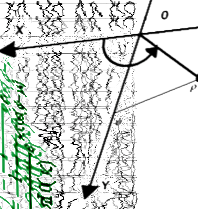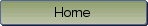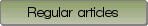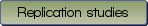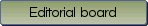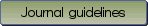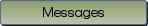A Tutorial on Testing the Equality of Standardized Regression Coefficients in Structural Equation Models using {W}ald Tests with lavaan

Bibliographic information: BibTEX format RIS format XML format APA style
Cited references information: BibTEX format APA style

Klopp, Eric
315-333
Keywords: comparing standardized regression coefficients , Wald test , structural equation modeling
Tools: lavaan
(no sample data)   (Appendix)

Comparing the effects of two or more explanatory variables on a dependent variable in structural equation models, with either manifest or latent variables, may be hampered by the arbitrary metrics which are common in social sciences and psychology. A possible way to compare the effects is the comparison of standardized regression coefficients by means of the Wald test. In this tutorial, we show how the Wald test can be used to compare standardized regression coefficients. Moreover, we demonstrate how this can be implemented in R using the lavaan package. Additionally, we provide a convenience function that allows doing a Wald test by only setting up equality constraints. The Wald test, its application and the implementation in R are illustrated by means of worked-examples. We also discuss theoretical aspects and implications when hypotheses about the equality of standardized regression parameters in structural equation models are tested.

Be informed of the upcoming issues with RSS feed:RSS